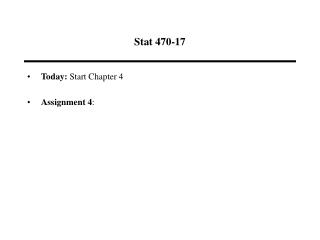Download PresentationStat 470-17

# Stat 470-17

Download Presentation## Stat 470-17

- - - - - - - - - - - - - - - - - - - - - - - - - - - E N D - - - - - - - - - - - - - - - - - - - - - - - - - - -
##### Presentation Transcript

1. Stat 470-17 • Today: Start Chapter 4 • Assignment 4:

2. Additional Features • Main effect or two-factor interactions (2fi) is clear if it is not aliased with other main effects, 2fi’s or block effects • Main effect or 2fi is strongly clear if it is not aliased with other main effects, 2fi’s, 3fi’s or block effects • As before, block by factor interactions are negligible • Analysis is same as before • Appendix 4 has blocked fractional factorial designs ranked by number of clear effects

3. Fractional Factorial Split-Plot Designs • It is frequently impractical to perform the fractional factorial design in a completely randomized manner • Can run groups of treatments in blocks • Sometimes the restrictions on randomization take place because some factors are hard to change or the process takes place in multiple stages • Fractional factorial split-plot (FFSP) design may be a practical option

4. Performing FFSP Designs • Randomization of FFSP designs different from fractional factorial designs • Have hard to change factors (whole-plot or WP factors) and easy to change factors (sub-plot or SP factors) • Experiment performed by: • selecting WP level setting, at random. • performing experimental trials by varying SP factors, while keeping the WP factors fixed.

5. Example • Would like to explore the impact of 6 factors in 16 trials • The experiment cannot be run in a completely random order because 3 of the factors (A,B,C) are very expensive to change • Instead, several experiment trials are performed with A, B, and C fixed…varying the levels of the other factors

6. Design Matrix

7. Impact of the Randomization Restrictions • Two Sources of randomization  Two sources of error • Between plot error: ew (WP error) • Within plot error: (SP error) • Model: • The WP and SP error terms have mutually independent normal distributions with standard deviations σw and σs

8. The Design • Situation: • Have k factors: k1 WP factors and k2 SP factors • Wish to explore impact in 2k-p trials • Have a 2k1-p1fractional factorial for the WP factors • Require p=p1+p2 generators • Called a 2(k1+ k2)-(p1+ p2) FFSP design

9. Constructing the Design • For a 2(k1+ k2)-(p1+ p2) FFSP design, have generators for WP and SP designs • Rules: • WP generators (e.g., I=ABC ) contain ONLY WP factors • SP generators (e.g., I=Apqr ) must contain AT LEAST 2 SP factors • Previous design: I=ABC=Apqr=BCpqr

10. Analysis of FFSP Designs • Two Sources of randomization  Two sources of error • Between plot error: σw (WP error). • Within plot error: σs (SP error). • WP Effects compared to: aσs2+ bσs2 • SP effects compared to : bσs2 • df for SP > df for WP • Get more power for SP effects!!!

11. Analysis of FFSP Designs • Variance of a WP effect • Variance of a SP effect

12. WP Effect or SP Effect? • Effects aliased with WP main effects or interactions involving only WP factors tested as a WP effect • E.g., pq=ABCD tested as a WP effect • Effects aliased only with SP main effects or interactions involving at least one SP factors tested as a SP effect • E.g., pq=ABr tested as a SP effect

13. Ranking the Designs • Use minimum aberration (MA) criterion

14. Example • Experiment is performed to study the geometric distortion of gear drives • The response is “dishing” of the gear • 5 factors thought to impact response: • A: Furnace track • B: Tooth size • C: Part position • p: Carbon potential • q: Operating Mode

15. Example • Because of the time taken to change the levels of some of the factors, it is more efficient to run experiment trials keeping factors A-C fixed and varying the levels of p and q • A 2(3+2)-(0+1) FFSP design was run ( I=ABCpq )

16. Example

17. Example • This is a 16-run design…have 15 effects to estimate • Which effects are test as WP effects? SP effects? • MA design: I=ABCpq • Have a 23 design for the WP effects: A, B, C, AB, AC, BC, ABC=pq are tested as WP effects • SP effects: p, q, Ap, Aq, Bp, Bq, Cp, Cq • Need separate qq-plots for each set of effects because they have different variances

18. QQ-Plots